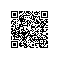# 【cocos2d-x从c++到js】05：John Resiq的继承写法解析

1. cc.Class = function(){};
2. cc.Class.extend = function (prop) {
3.     var _super = this.prototype;
4.
5.     // Instantiate a base class (but only create the instance,
6.     // don't run the init constructor)
7.     initializing = true
8.     var prototype = new this();
9.     initializing = false
10.     fnTest = /xyz/.test(function(){xyz;}) ? /\b_super\b/ : /.*/;
11.
12.     // Copy the properties over onto the new prototype
13.     for (var name in prop) {
14.         // Check if we're overwriting an existing function
15.         prototype[name] = typeof prop[name] == "function" &&
16.             typeof _super[name] == "function" && fnTest.test(prop[name]) ?
17.             (function (name, fn) {
18.                 return function () {
19.                     var tmp = this._super;
20.
21.                     // Add a new ._super() method that is the same method
22.                     // but on the super-class
23.                     this._super = _super[name];
24.
25.                     // The method only need to be bound temporarily, so we
26.                     // remove it when we're done executing
27.                     var ret = fn.apply(this, arguments);
28.                     this._super = tmp;
29.
30.                     return ret;
31.                 };
32.             })(name, prop[name]) :
33.             prop[name];
34.     }
35.
36.     // The dummy class constructor
37.     function Class() {
38.         // All construction is actually done in the init method
39.         if (!initializing && this.ctor)
40.             this.ctor.apply(this, arguments);
41.     }
42.
43.     // Populate our constructed prototype object
44.     Class.prototype = prototype;
45.
46.     // Enforce the constructor to be what we expect
47.     Class.prototype.constructor = Class;
48.
49.     // And make this class extendable
50.     Class.extend = arguments.callee;
51.
52.     return Class;
53. };

1. cc.Class = function(){};

1. cc.Class.extend = function (prop) {

prop是一个对象字面量，这个对象包含了子类所需要的全部成员变量和成员方法。extend函数就在内部遍历这个字面量的属性，然后将这些属性绑定到一个“新的构造函数”（也就是子类的构造函数）的原型上。

1. var _super = this.prototype;

1. var MyNode = cc.Node.extend({
2. var _super = this.prototype;
3. ...
4. });

1. initializing = true
2. var prototype = new this();
3. initializing = false

1. fnTest = /xyz/.test(function(){xyz;}) ? /\b_super\b/ : /.*/;

1.  for (var name in prop) {
2.         prototype[name] = typeof prop[name] == "function" &&
3.             typeof _super[name] == "function" && fnTest.test(prop[name]) ?
4.             (function (name, fn) {
5.                 return function () {
6.                     var tmp = this._super;
7.                     this._super = _super[name];
8.                     var ret = fn.apply(this, arguments);
9. this._super = tmp;
10.                     return ret;
11.                 };
12.             })(name, prop[name]) :
13.             prop[name];
14. }

1. typeof prop[name] == "function" && typeof _super[name] == "function"

1. (function (name, fn) {
2.     return function () {
3.         var tmp = this._super;
4.         this._super = _super[name];
5.         var ret = fn.apply(this, arguments);
6.         this._super = tmp;
7.         return ret;
8.     };
9. })(name, prop[name])

1. function Class() {
2.    if (!initializing && this.ctor)
3.         this.ctor.apply(this, arguments);
4. }
5.
6. Class.prototype = prototype;
7. Class.prototype.constructor = Class;
8. Class.extend = arguments.callee;

本文转自 老G 51CTO博客，原文链接：http://blog.51cto.com/goldlion/1127020，如需转载请自行联系原作者使用钉钉扫一扫加入圈子
+ 订阅